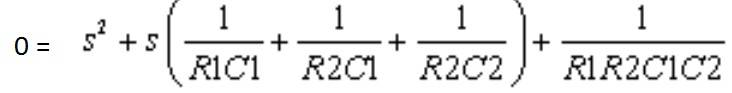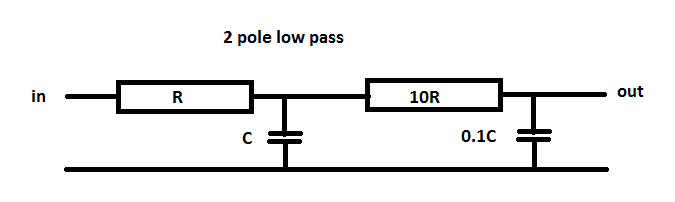# 2nd order RC filter with two equal poles

Is it possible to make a 2nd order CR LPF or HPF where the cut off frequencies for each pole are equal?

Here is a calculator for this system which includes the transfer function.http://sim.okawa-denshi.jp/en/CRCRkeisan.htm

I figured that I need to try to solve the denominator of the transfer function in this way and impose the restriction that both roots of S must be equal, but I'm not sure how to do that.NascentOxygen
Staff Emeritus
In principle, you can set the poles equal.

Are you sure a passive filter will do the job? The source has low resistance and you won't have loading on the filter output? The filter shape doesn't matter much?

It's going to be implemented between two discrete BJT amplifier stages. It's a filter within an amplifier basically, so yeah there's not going to be a loss of signal.

NascentOxygen
Staff Emeritus
The BJT input loads the filter output and changes the response.

Yeah I know. My output and input impedances will be adjusted to account for this.

NascentOxygen
Staff Emeritus
My output and input impedances will be adjusted to account for this.

I'm beginning to think that two poles at the same positions isn't possible.
My reasoning is that for them to be the same, they're going to be a complex conjugate pair.

And for that to be true, if we look at the standard quadratic formula we see that
4ac needs to be greater than b^2

And using the transfer function I posted to obtain b,a, and c
I performed the following analysis at wolfram

http://www.wolframalpha.com/input/?i=4*(1/abcd)>((1/ac)+(1/bc)+(1/bd))^2

and you can see that some of the resistor and capacitor values end up needing to be negative

NascentOxygen
Staff Emeritus
I wasn't referring to varying load. If the filter is to work into a fixed load, then that load needs to feature in the filter equation.

okay, so even though it's not possible to have two equal poles with the transfer function I posted, maybe it's possible if the load is taken into account. I will have to formulate that transfer function and check. Also, there's a different post above to the one I initially posted if you missed it.

Is it possible to make a 2nd order CR LPF or HPF where the cut off frequencies for each pole are equal?
A second-order high- or lowpass filter with a double-pole (two equal poles on the neg. real axis of the s-plane) is possible only if both filter blocks are separated by a buffer amplifier (no loading of the first stage by the second stage).
In this case, the pole quality factor will be 0.5.
In this case, the term R2C1 disappears in the denominator.
Any transistor-based buffer amplifier will do the job of buffering only approximately because of finite input and output impedances, unless you include these impedances in the calculation of the filter elements.

Last edited:
A second-order high- or lowpass filter with a double-pole (two equal poles on the neg. real axis of the s-plane) is possible only if both filter blocks are separated by a buffer amplifier (no loading of the first stage by the second stage).
In this case, the pole quality factor will be 0.5.
Any transistor-based buffer amplifier will do the job of buffering only approximately because of finite input and output impedances, unless you include these impedances in the calculation of the filter elements.

Yeah, I thought this was probably what I would have to opt towards doing, I just wanted to make sure.

If you have gain to spare, you can put matched pads, 10dB or so, between the poles.

jim hardy
Gold Member
Dearly Missed
Rule of thumb